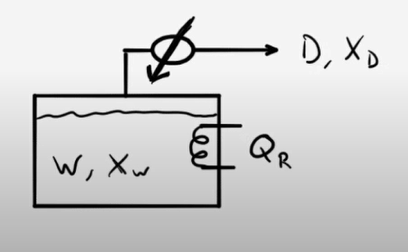LearnChemE

#### Single-Stage Batch Distillation: Example Problems

Try to solve these problems before watching the solutions in the screencasts.

##### Example Problem 1

50 moles of an ethanol-water mixture are loaded into a pot still and heated. When the first distillate is collected, the head temperature (vapor temperature) is 84°C. The still is run until the head temperature is 89°C. What is the total amount of distillate collected? What are the compositions of the distillate and the waste products?##### Example Problem 2

A single-stage batch still operating at 760 mm Hg pressure is charged with 75 mol of a 82 mol% methanol(1)/18 mol% water(2) mixture. Assume the mixture is an ideal solution.
How much distillate will be collected if the average distillate concentration desired is 90.0%?
How many moles will remain in the still and what is its methanol concentration?
The Antione equations (where $$T$$ is in Celsius) are:

Methanol:   $$log_{10}P_1^{sat}\,=\,8.081-\left(\frac{1582}{T+239.7}\right)$$

Water:   $$log_{10}P_2^{sat}\,=\,8.071-\left(\frac{1731}{T+233.4}\right)$$